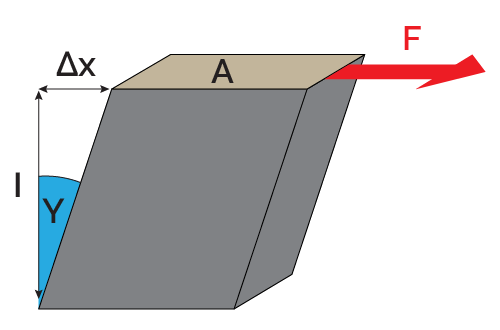# Shear Modulus Calculator

Created by Luis Hoyos
Last updated: Nov 16, 2022

With this shear modulus calculator, you'll be able to calculate the modulus of rigidity (shear modulus), a quantity required for calculating shear strain, and mechanical vibrations.

You can use this modulus of rigidity calculator in two ways:

• Obtain the shear modulus by inputting the area (A), length (l), and displacement (Δx) of a cubic element subjected to a force F; or
• Calculate the modulus of rigidity by inputting the shear stress (𝜏) and shear strain (γ).

If you want to learn more, in the rest of this article, we present the formulas and the shear modulus of aluminum and many other materials.

## What is the shear modulus?

In engineering, the shear modulus indicates the stiffness or resistance to deformation under shear stress. By definition, it is the ratio of shear stress to the shear strain, a definition that applies as long as the material behaves in a linear elastic way.

The shear modulus is one of several quantities derived from stress-strain measurements that we can use to characterize elastic materials. Another similar quantity is the widely known Young's modulus, which we can calculate similarly using our Young's modulus calculator. In fact, all the elastic materials' properties relate to each other, as seen in our Poisson's ratio calculator, which allows calculating that property by inputting the Young's and shear modulus.

Along with the polar moment of inertia and shaft length, the shear modulus is a quantity we can also use in the mechanics of materials to obtain the angle of twist of shafts subjected to torsional loads (an indicator of shear strain).

## Shear modulus formula

By definition, the shear modulus of a material is the ratio of the shear stress applied to it to the shear strain occasionated:

G = 𝜏/γ

, where:

• G — Shear modulus;
• 𝜏 — Shear stress; and
• γ — Shear strain.

Another way to calculate the shear modulus is by defining a cubic element over which a force (tangent to its surface) acts.

From the shear stress definition ($\tau = F/A$) and the shear strain definition ($\gamma = \Delta x/l$), we can obtain the following shear modulus equation:

$G = \frac{Fl}{A \Delta x}$

, where:

• $F$ — Force magnitude;
• $A$ — Area over which the force acts;
• $l$ — Deformation or displacement caused by the force $F$; and
• $\Delta x$ — Length of the cubic element transverse to the displacement.

We use both of the equations in the shear modulus calculator so you can quickly use it to find the answer you're looking for.

## Shear modulus of aluminum and other materials

If you already know the material, you don't need to calculate the modulus of rigidity with the previous shear modulus equations, but only take a look at the following list of shear moduli:

• Aluminum: 25 GPa (3.63 × 10⁶ psi);
• Brass: 35 GPa (5.08 × 10⁶ psi);
• Copper: 44 GPa (6.38 × 10⁶ psi);
• Iron: 77 GPa (11.2 × 10⁶ psi);
• Lead: 6 GPa (0.87 × 10⁶ psi);
• Silicone rubber: 0.002 GPa (0.00029 × 10⁶ psi); and
• Steel: 75 GPa (10.9 × 10⁶ psi);
Luis HoyosInput F, A, l, and Δx
Force magnitude (F)
lbf
Area over which the force acts (A)
ft²
Transverse length (l)
ft
Displacement (Δx)
ft
or input 𝜏 and γ
Shear stress (𝜏 = F/A)
psi
Shear strain (γ ≈ Δx/L)
Shear modulus (G)
psi
People also viewed…

### Bend allowance

Use the bend allowance calculator to find the bend allowance and deduction of a metal sheet.

### Brinell hardness number

Calculate a material's indentation hardness with the Brinell hardness number calculator.